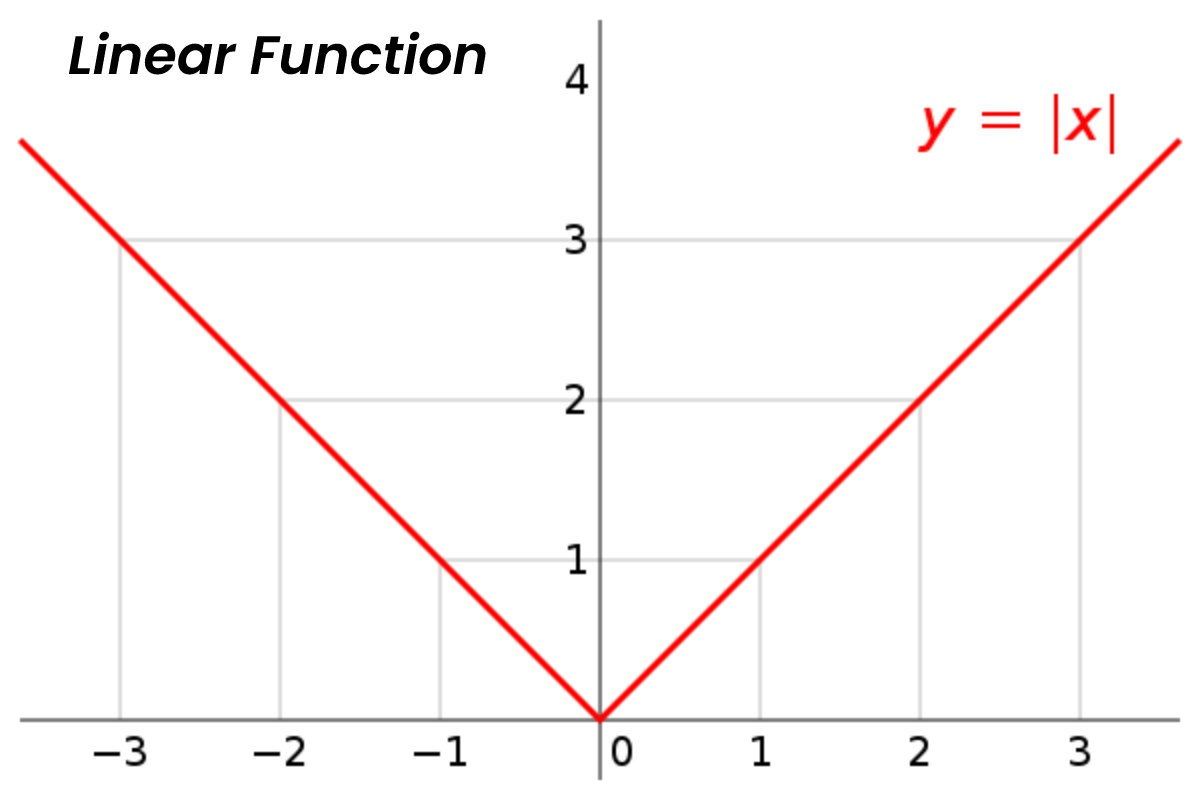# Linear Function – An Integral Part of MathematicsWellness

Linear Function – Mathematics is one of the oldest topics. Math is also considered one of the most important subjects in student life. Mathematics has had a tremendous impact on how our environment has changed. Mathematical applications are found in almost every aspect of our life. Some of the best mathematicians in the world have solved some of the world’s most challenging problems, and left their mark on the discipline of mathematics. Maths is one of those subjects that are studied by everyone irrespective of their stream, whether one is from arts, commerce or science background. It is a topic with a wide range of applications in real-world situations.

The use of mathematics cannot be ignored in any field. In coding maths is a critical subject, to manage the stock market and finance one should be clear with their concepts of math. It is critical to understand the fundamentals of mathematics. Even in the current educational curriculum, mathematics is the most significant subject, which is a crucial component of remarkable fields. If one can pay attention to it and practise all types of problems, this difficult subject can be quite entertaining. A few of the major topics of mathematics are geometry, algebra, statics and calculus. One such topic that has huge importance in the field of mathematics is function. There are many types of functions in mathematics, one such function is a linear function. A linear function is considered an algebraic equation in which every term is either a constant or the product of a constant and a single variable.

Anyone who has once in a life has studied math must have heard the word functions. Linear functions are used in almost every topic of math. It doesn’t matter whether it’s algebra geometry or calculus. There are many different types of functions. A linear function is one such type of function. Linear functions consist of only one variable. Here we are going to discuss linear function in detail.

• Linear function: Any equation that is of the form of f(x)= ax+b is said to be a linear function, where a and b are constants, a should not be equal to zero and also x is variable. If a is constant in the above equation, then it is not treated as a linear function; it is then treated as constant. People should keep in mind that the domain of a linear function always consists of real numbers. Similarly, the range of a linear function also consists of all the real numbers. When a linear function is plotted on a graph, it comes out to be a straight line whose slope is a constant term. Graph of a linear function is always a straight line but a vertical line is not the graph of a function. To find a solution to any function, we need as many equations as there are the number of variables. In a linear equation, as there is only one variable, we need only one equation to find its solution. We apply linear functions in our everyday life for solving an equation.

In the previous post, we attempted to cover all of the concepts connected to linear functions. Linear functions find a lot of application in the real world. Because of the rise of online learning, people may now find a range of venues to get information. One such platform is Cuemath. It’s one of the most effective methods for making our math problems clear. It is written in an easy-to-understand language. On it, you can read about a variety of math-related topics.

This platform may assist everyone of any age, not just school or college students, by providing access to a large amount of information available. The popularity of online learning has soared in recent years. One of the benefits of these platforms is that people can study from these platforms in the comfort of their homes. People should take advantage of such a platform.